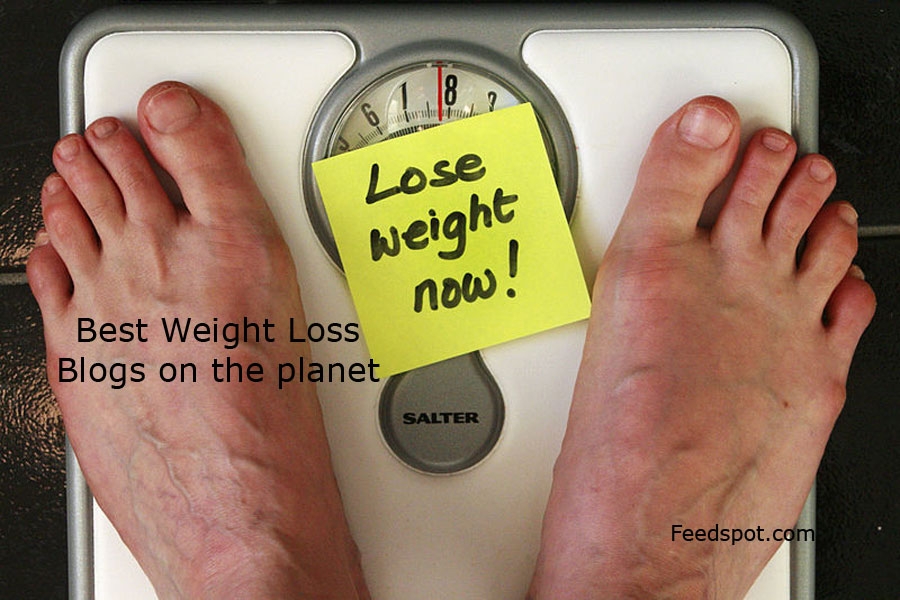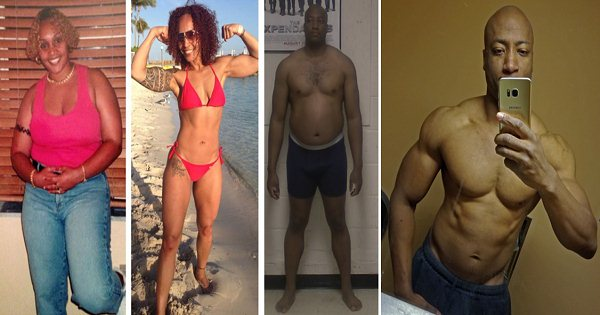!function(n,t){function r(e,n){return Object.prototype.hasOwnProperty.call(e,n)}function i(e){return void 0===e}if(n){var o={},s=n.TraceKit,a=[].slice,l="?";o.noConflict=function(){return n.TraceKit=s,o},o.wrap=function(e){function n(){try{return e.apply(this,arguments)}catch(e){throw o.report(e),e}}return n},o.report=function(){function e(e){l(),h.push(e)}function t(e){for(var n=h.length-1;n>=0;--n)h[n]===e&&h.splice(n,1)}function i(e,n){var t=null;if(!n||o.collectWindowErrors){for(var i in h)if(r(h,i))try{h[i].apply(null,[e].concat(a.call(arguments,2)))}catch(e){t=e}if(t)throw t}}function s(e,n,t,r,s){var a=null;if(w)o.computeStackTrace.augmentStackTraceWithInitialElement(w,n,t,e),u();else if(s)a=o.computeStackTrace(s),i(a,!0);else{var l={url:n,line:t,column:r};l.func=o.computeStackTrace.guessFunctionName(l.url,l.line),l.context=o.computeStackTrace.gatherContext(l.url,l.line),a={mode:"onerror",message:e,stack:[l]},i(a,!0)}return!!f&&f.apply(this,arguments)}function l(){!0!==d&&(f=n.onerror,n.onerror=s,d=!0)}function u(){var e=w,n=p;p=null,w=null,m=null,i.apply(null,[e,!1].concat(n))}function c(e){if(w){if(m===e)return;u()}var t=o.computeStackTrace(e);throw w=t,m=e,p=a.call(arguments,1),n.setTimeout(function(){m===e&&u()},t.incomplete?2e3:0),e}var f,d,h=[],p=null,m=null,w=null;return c.subscribe=e,c.unsubscribe=t,c}(),o.computeStackTrace=function(){function e(e){if(!o.remoteFetching)return"";try{var t=function(){try{return new n.XMLHttpRequest}catch(e){return new n.ActiveXObject("Microsoft.XMLHTTP")}},r=t();return r.open("GET",e,!1),r.send(""),r.responseText}catch(e){return""}}function t(t){if("string"!=typeof t)return[];if(!r(j,t)){var i="",o="";try{o=n.document.domain}catch(e){}var s=/(.*)\:\/\/([^:\/]+)([:\d]*)\/{0,1}([\s\S]*)/.exec(t);s&&s===o&&(i=e(t)),j[t]=i?i.split("\n"):[]}return j[t]}function s(e,n){var r,o=/function ([^(]*)\(([^)]*)\)/,s=/['"]?([0-9A-Za-z\$_]+)['"]?\s*[:=]\s*(function|eval|new Function)/,a="",u=10,c=t(e);if(!c.length)return l;for(var f=0;f0?s:null}function u(e){return e.replace(/[\-\[\]{}()*+?.,\\\^\$|#]/g,"\\\$&")}function c(e){return u(e).replace("<","(?:<|<)").replace(">","(?:>|>)").replace("&","(?:&|&)").replace('"','(?:"|")').replace(/\s+/g,"\\s+")}function f(e,n){for(var r,i,o=0,s=n.length;or&&(i=s.exec(o[r]))?i.index:null}function h(e){if(!i(n&&n.document)){for(var t,r,o,s,a=[n.location.href],l=n.document.getElementsByTagName("script"),d=""+e,h=/^function(?:\s+([\w\$]+))?\s*\(([\w\s,]*)\)\s*\{\s*(\S[\s\S]*\S)\s*\}\s*\$/,p=/^function on([\w\$]+)\s*\(event\)\s*\{\s*(\S[\s\S]*\S)\s*\}\s*\$/,m=0;m]+)>|([^\)]+))\((.*)\))? in (.*):\s*\$/i,o=n.split("\n"),l=[],u=0;u=0&&(g.line=v+x.substring(0,j).split("\n").length)}}}else if(o=d.exec(i[y])){var _=n.location.href.replace(/#.*\$/,""),T=new RegExp(c(i[y+1])),E=f(T,[_]);g={url:_,func:"",args:[],line:E?E.line:o,column:null}}if(g){g.func||(g.func=s(g.url,g.line));var k=a(g.url,g.line),A=k?k[Math.floor(k.length/2)]:null;k&&A.replace(/^\s*/,"")===i[y+1].replace(/^\s*/,"")?g.context=k:g.context=[i[y+1]],h.push(g)}}return h.length?{mode:"multiline",name:e.name,message:i,stack:h}:null}function y(e,n,t,r){var i={url:n,line:t};if(i.url&&i.line){e.incomplete=!1,i.func||(i.func=s(i.url,i.line)),i.context||(i.context=a(i.url,i.line));var o=/ '([^']+)' /.exec(r);if(o&&(i.column=d(o,i.url,i.line)),e.stack.length>0&&e.stack.url===i.url){if(e.stack.line===i.line)return!1;if(!e.stack.line&&e.stack.func===i.func)return e.stack.line=i.line,e.stack.context=i.context,!1}return e.stack.unshift(i),e.partial=!0,!0}return e.incomplete=!0,!1}function g(e,n){for(var t,r,i,a=/function\s+([_\$a-zA-Z\xA0-\uFFFF][_\$a-zA-Z0-9\xA0-\uFFFF]*)?\s*\(/i,u=[],c={},f=!1,p=g.caller;p&&!f;p=p.caller)if(p!==v&&p!==o.report){if(r={url:null,func:l,args:[],line:null,column:null},p.name?r.func=p.name:(t=a.exec(p.toString()))&&(r.func=t),"undefined"==typeof r.func)try{r.func=t.input.substring(0,t.input.indexOf("{"))}catch(e){}if(i=h(p)){r.url=i.url,r.line=i.line,r.func===l&&(r.func=s(r.url,r.line));var m=/ '([^']+)' /.exec(e.message||e.description);m&&(r.column=d(m,i.url,i.line))}c[""+p]?f=!0:c[""+p]=!0,u.push(r)}n&&u.splice(0,n);var w={mode:"callers",name:e.name,message:e.message,stack:u};return y(w,e.sourceURL||e.fileName,e.line||e.lineNumber,e.message||e.description),w}function v(e,n){var t=null;n=null==n?0:+n;try{if(t=m(e))return t}catch(e){if(x)throw e}try{if(t=p(e))return t}catch(e){if(x)throw e}try{if(t=w(e))return t}catch(e){if(x)throw e}try{if(t=g(e,n+1))return t}catch(e){if(x)throw e}return{mode:"failed"}}function b(e){e=1+(null==e?0:+e);try{throw new Error}catch(n){return v(n,e+1)}}var x=!1,j={};return v.augmentStackTraceWithInitialElement=y,v.guessFunctionName=s,v.gatherContext=a,v.ofCaller=b,v.getSource=t,v}(),o.extendToAsynchronousCallbacks=function(){var e=function(e){var t=n[e];n[e]=function(){var e=a.call(arguments),n=e;return"function"==typeof n&&(e=o.wrap(n)),t.apply?t.apply(this,e):t(e,e)}};e("setTimeout"),e("setInterval")},o.remoteFetching||(o.remoteFetching=!0),o.collectWindowErrors||(o.collectWindowErrors=!0),(!o.linesOfContext||o.linesOfContext<1)&&(o.linesOfContext=11),void 0!==e&&e.exports&&n.module!==e?e.exports=o:"function"==typeof define&&define.amd?define("TraceKit",[],o):n.TraceKit=o}}("undefined"!=typeof window?window:global)},"./webpack-loaders/expose-loader/index.js?require!./shared/require-global.js":function(e,n,t){(function(n){e.exports=n.require=t("./shared/require-global.js")}).call(n,t("../../../lib/node_modules/webpack/buildin/global.js"))}});```"Researchers around the world say what really works is not just cutting calories but satisfying your hunger with the right kinds of foods," says Health’s Frances Largeman-Roth, RD. In fact, women following a low-fat diet who were allowed to fill up on all the fruit and vegetables they wanted lost 23% more weight than women on a low-fat diet alone, a new study from the United Kingdom reports.```

```While there are probably plenty of pre-made bean and veggie soup options that just need a few minutes to heat through on the stovetop, making your own soup is really easy—and a great idea for your health. Homemade soups are much lower in sodium – about 100 milligrams or less per 2-cup serving. By contrast, 2 cups of many canned soups contain a blood-pressure-busting 1,200 milligrams or more, a worrisome amount considering that health experts recommend consuming no more than 1,500 milligrams of sodium for the entire day. This is also a great way to use up all those leftover vegetables in your crisper—pretty much anything works in this soup.```

Purchase an electric teakettle. Electric teakettles are readily available at many bath and kitchen stores, ranging in price and are extremely easy to use. All you have to do is fill it with water and push a button or lever to bring it to a boil. You can brew tea by the cup or add several tea bags to the entire pot once the water has boiled. Keep a thermos as well for the additional boiled water. Fill with water, add the green tea and keep by the kettle or your desk for ease of pouring a tea when needed.The current study showed that both protocols of WL could improve components of the lipid and glycemic profiles. In addition, in this study it was found that with the same amount of WL, the impact on reducing levels of FBS and LDL, and improvement of insulin resistance and sensitivity was greater with rapid WL. Positive effects of rapid WL on metabolic factors were reported in several studies.
Your favorite childhood snack is good for your grown-up self, too. Because peanut butter is a great source of protein and healthy fats, it can curb hunger and keep you feeling full long after you're finished eating. Having small snacks during the day that include a lean protein such as peanut butter with complex carbs (like an apple or banana) can also help keep your metabolism running smoothly.
Lipton green tea is loaded with epigallocatechin gallate (EGCG), a catechin that is found in green tea. It helps your body to burn fat and increases your body’s insulin sensitivity (3). EGCG has also been found to inhibit fatty liver disease in lab rats (4). If you are a beginner, then the green tea containing honey and pomegranate is a better option for weight loss. But all the variants have the same impact on your body.```Salmon boasts significant anti-inflammatory properties thanks to its rich omega-3 fatty acid content, meaning it’s an excellent source of protein for those looking to jumpstart their weight loss. In fact, one study that examined the effects of weight loss and seafood consumption showed wild salmon to be the most effective at reducing inflammation—better than lean white fish and a fish-free diet. According to a study published in The Journal of Nutritional Biochemistry, fishy fatty acids may also signal thyroid cells in the liver to burn more fat.
```

Thank you for this meal plan. It is exactly what I needed and having the shopping list was great. It made me see that I needed to cut portions, eat better, and skip or significantly moderate sweets and alcohol. I have made some minor substitutions like doubling broccoli because I don’t like Brussels sprouts, but for the most part sticking to the plan. I expected to feel hungry and don’t with the snacks.Identifying and resolving typical weight loss hurdles — like flagging enthusiasm — is something Noom excels at. The initial questions that set up your profile, match you with a coach, and place you with a group, all intend to diagnose your learning style and what flavor of support you need. What kicks you into high gear, tough love or words of affirmation? How do you like to show support? How do you feel about goal setting?
Another win for your morning cup of joe: Caffeinated coffee keeps things moving through the digestive tract. Since staying regular is key to a tighter-looking tummy, drinking about 8 to 16 ounces of java at the same time every day can help you stay on schedule. Remember: Sugary drinks can lead to weight gain, so skip fancy flavorings and synthetic sweeteners containing sugar alcohols, which can cause bloating.

Losing weight doesn’t always have to be about deprivation and denial. In fact, it shouldn’t be. Successful, sustainable weight loss is far more attainable when you focus on the quality of food rather than the quantity. Eat wholesome, nutritious, (and even calorie-filled) foods and you’ll be far more satisfied and content on less. Many of the foods people think are off-limits when it comes to losing weight are the very foods that have the ability to actually help us reach our goal. Here are eight foods that cannot only help you reach your weight-loss goal, but help you keep it off for good.
Grains get a bad rap when it comes to weight loss, but that's because refined grains (read: processed foods!) are linked to wider waists. 100% whole grains are bloat-busting superstars, however, as they're packed with minerals and de-puff by counter-balancing salt. Stick to brown rice, quinoa, wheat, barley, millet, farro, sorghum, and amaranth for the biggest benefits.
By now, you probably know that coffee can help curb your appetite. But did you also know it can boost your metabolism? Since coffee contains the antioxidant chlorogenic acid (CGA), it can actually increase your body’s use of fat for energy. Research has additionally shown that CGA can slow the release of glucose and lower insulin resistance to inhibit weight gain after eating a meal.!function(e){function n(t){if(r[t])return r[t].exports;var i=r[t]={i:t,l:!1,exports:{}};return e[t].call(i.exports,i,i.exports,n),i.l=!0,i.exports}var t=window.webpackJsonp;window.webpackJsonp=function(n,r,o){for(var s,a,l=0,u=[];l1)for(var t=1;tf)return!1;if(h>c)return!1;var e=window.require.hasModule("shared/browser")&&window.require("shared/browser");return!e||!e.opera}function a(){var e=o(d);d=[],0!==e.length&&u("/ajax/log_errors_3RD_PARTY_POST",{errors:JSON.stringify(e)})}var l=t("./third_party/tracekit.js"),u=t("./shared/basicrpc.js").rpc;l.remoteFetching=!1,l.collectWindowErrors=!0,l.report.subscribe(r);var c=10,f=window.Q&&window.Q.errorSamplingRate||1,d=[],h=0,p=i(a,1e3),m=window.console&&!(window.NODE_JS&&window.UNIT_TEST);n.report=function(e){try{m&&console.error(e.stack||e),l.report(e)}catch(e){}};var w=function(e,n,t){r({name:n,message:t,source:e,stack:l.computeStackTrace.ofCaller().stack||[]}),m&&console.error(t)};n.logJsError=w.bind(null,"js"),n.logMobileJsError=w.bind(null,"mobile_js")},"./shared/globals.js":function(e,n,t){var r=t("./shared/links.js");(window.Q=window.Q||{}).openUrl=function(e,n){var t=e.href;return r.linkClicked(t,n),window.open(t).opener=null,!1}},"./shared/links.js":function(e,n){var t=[];n.onLinkClick=function(e){t.push(e)},n.linkClicked=function(e,n){for(var r=0;r>>0;if("function"!=typeof e)throw new TypeError;for(arguments.length>1&&(t=n),r=0;r>>0,r=arguments.length>=2?arguments:void 0,i=0;i>>0;if(0===i)return-1;var o=+n||0;if(Math.abs(o)===Infinity&&(o=0),o>=i)return-1;for(t=Math.max(o>=0?o:i-Math.abs(o),0);t>>0;if("function"!=typeof e)throw new TypeError(e+" is not a function");for(arguments.length>1&&(t=n),r=0;r>>0;if("function"!=typeof e)throw new TypeError(e+" is not a function");for(arguments.length>1&&(t=n),r=new Array(s),i=0;i>>0;if("function"!=typeof e)throw new TypeError;for(var r=[],i=arguments.length>=2?arguments:void 0,o=0;o>>0,i=0;if(2==arguments.length)n=arguments;else{for(;i=r)throw new TypeError("Reduce of empty array with no initial value");n=t[i++]}for(;i>>0;if(0===i)return-1;for(n=i-1,arguments.length>1&&(n=Number(arguments),n!=n?n=0:0!==n&&n!=1/0&&n!=-1/0&&(n=(n>0||-1)*Math.floor(Math.abs(n)))),t=n>=0?Math.min(n,i-1):i-Math.abs(n);t>=0;t--)if(t in r&&r[t]===e)return t;return-1};t(Array.prototype,"lastIndexOf",c)}if(!Array.prototype.includes){var f=function(e){"use strict";if(null==this)throw new TypeError("Array.prototype.includes called on null or undefined");var n=Object(this),t=parseInt(n.length,10)||0;if(0===t)return!1;var r,i=parseInt(arguments,10)||0;i>=0?r=i:(r=t+i)<0&&(r=0);for(var o;rweight loss foods